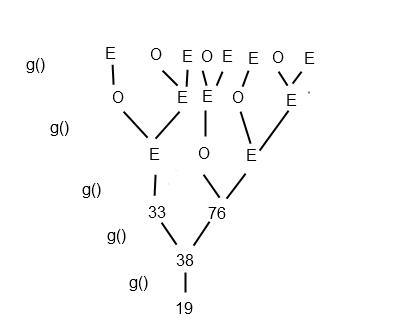# (GMAT 800)The function g(x) is defined for integers x such that if x

The function g(x) is defined for integers x such that if x is even, g(x) = x/2 and if x is odd, g(x) = x + 5.  Given that g(g(g(g(g(x))))) = 19, how many possible values for x would satisfy this equation?

A. 1
B. 5
C. 7
D. 8
E. 11

Explanation:

The easiest way to approach this problem is probably to work backwards, at least until we see a pattern.

With g(...) = 19, then we can consider which operation applied to (...).  If it was x/2, then (...)= 38.  38 is even so that is fair.   If it was x + 5, then (...) was 14.  14 is even, so that operation would not have been applied.

On paper, you could make a tree, with 19 as the root, and 38 as the first node.

Next consider 38.  38 could have come from 76/2 or 33 + 5.  Two possibilities give us two nodes branching from 38:

19  -> 38 -> 76, 33.

We can now observe the pattern that with an odd number, it must have come from an even, but an even could come from either of two numbers.

Therefore our 76 will branch into 2 numbers, and the 33 into just one.

33 -> 66
76 -> 73, 152..

We can represent this as shown here:Counting at the base we see we have 8 original possibilities.Grockit,an online test  prep game, is the smartest way to study for your test. It's adaptive, fun and finds the right teacher for you. Grockit’s analytic capabilities and adaptive technology identifies students' strengths and weaknesses, focusing the student's study time.  Students can practice in adaptive solo games, play social learning games with peers, and work with experts that match their specific needs.1. Complete GMAT RC Questions in less than 1 minute and 50 seconds
3. Take Notes Effectively
4. Collect and Interpret Facts
5. Speed up Summary Creation
6. Remember Information
7. Question the Author

12. Learn to Answer GMAT organization of passage Question
13. Learn to identify the style/tone or attitude of the author

Mastering GMAT Critical ReasoningAfter you read F1GMAT’s Mastering GMAT Critical Reasoning Guide, you will learn:

How to overcome flawed thinking in GMAT Critical Reasoning?

How to spot Inconsistencies in Arguments

How to eliminate out of scope answer choices using Necessary and Sufficient Conditions

How to Paraphrase GMAT Critical Reasoning Question

How to Answer Assumption Question Type

How to Answer Conclusion Question Type

How to Answer Inference Question Type

How to Answer Strengthen Question Type

How to Answer Weaken Question Type

How to Answer bold-faced and Summary Question Types

How to Answer Parallel Reasoning Questions

How to Answer the Fill in the Blanks Question

Get F1GMAT's Newsletters (Best in the Industry)

• Ranking Analysis
• Post-MBA Salary Trends
• Post-MBA Job Function & Industry Analysis
• Post-MBA City Review
• MBA Application Essay Tips
• School Specific Essay Tips
• GMAT Preparation Tips